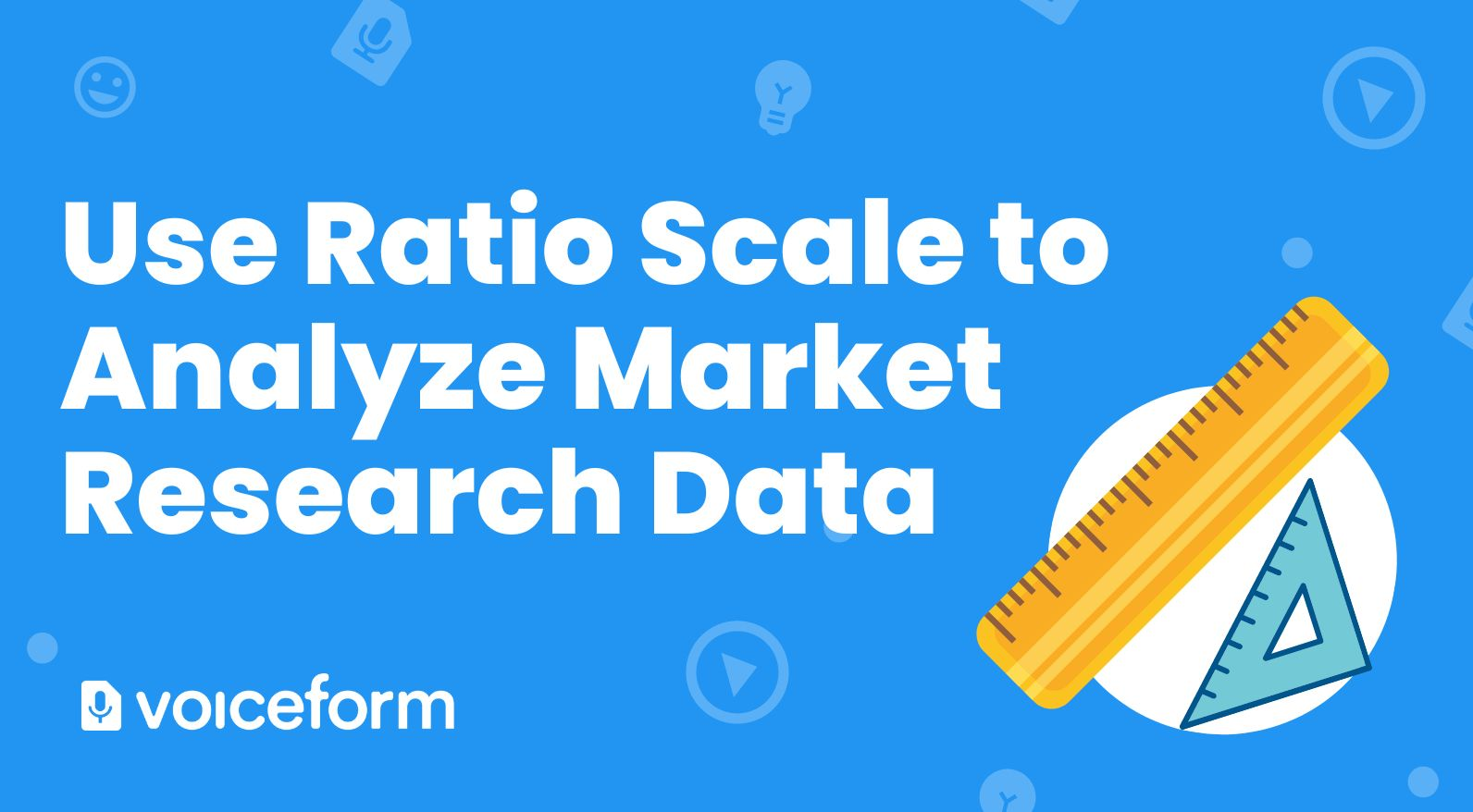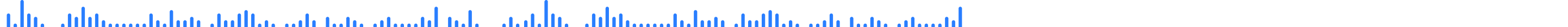# What is Ratio Scale? With 5 Excellent Examples

Learn what a ratio scale is and how to use it in market research analysis.When you’re collecting data for research purposes, you’ll probably group that data according to certain levels of measurement. Different types of data require different measurements. Ratio scales are one way to analyze and understand your research data. They apply to measurements in which there is a true zero value, making it easier to draw comparisons between variables like age, time, weight, and more.

This article will help you understand the ratio scale, as well as provide some ratio scale examples to help you understand the concept. Read on to find out what it is and what kind of measurements are suitable for this type of analysis.

## What is a ratio scale?

A ratio scale is a type of market research tool. It’s the highest ranked in the four levels of measurement, based on the precision involved. Ratio scales allow you to categorize and rank your data along equal intervals. The scale has a true zero and no negative values, allowing researchers to discover and describe the amount of magnitude involved. For example, length, money, age, duration, and mass can all be measured on a ratio scale. In market research questions, ratio scales are useful when analyzing concepts like sales numbers, market share, customer data, and pricing.

Ratio scale describes the order and the equal distance between two values. The variables can be added, subtracted, multiplied, and divided, and there are no negative values involved. For example, temperature can’t be measured on a ratio scale, because 0 degrees Celsius and Fahrenheit is not the “true zero point” for temperature. True zero is an absence of the variable. Temperature still exists at the zero point. Therefore, ratio scales are not appropriate to measure Celsius and Fahrenheit temperature. However, the Kelvin scale has a true zero—0k—which makes it suitable for ratio scale measurement.

A ratio scale allows analysts to convert units, like calories, speed, weight, joules, watts, and more. Finally, ratio scale allows both countable numbers and infinite values.

## Why use a ratio scale?

Ratio scales encompass four distinct levels of measurement:

1. Labels and categorizes variables (nominal)
2. Ranks categories available (ordinal)
3. Encompasses equal, known intervals (interval)
4. Has a true or meaningful zero (ratio)

This allows you to examine the relationship between the values assigned to any selected variable. For example, if your variable is the number of sales in the last three months, you’ll know that the value of 10 sales is double the value of five sales. This allows analysts to conduct complicated statistical analysis, depending on your research question and the variables involved.

Analysts can use ratio scale data by plotting it on graphs or grouping it in tables. It’s also possible to use mode, median, and/or mean to find your central tendencies. You can use your ratio scale data to find the range, variance, and standard deviation in the data, whether that’s number of sales and clients or customer age and income.

## Ratio scale examples

Practical ratio scale examples will help you understand the value of using this type of measurement.

### Miles per hour

Speed is an easy example of the ratio scale. Because there is a true zero value—the absence of forward motion—it’s easy to plot and visualize speed data. For instance, we know that 50 miles per hour is half the speed of 100 mph, and 200 mph is twice the speed of 100 mph and four times the speed of 50mph. This allows you to make comparisons between different variables and values to see how they relate to each other.

### Time

Time spent can be measured on a ratio scale, since “negative time” doesn’t exist. If you’re researching how long it takes customers to make a purchase on your website, you would measure from the moment they initiate the purchasing process to the final “place your order” command. Again, you know that 10 minutes is twice the value of five minutes, whereas an hour is 12 times the value of five minutes.

### Weight

Weight also has a true zero, making it appropriate for ratio scales. Whether you’re measuring in milligrams, kilograms, ounces, or pounds, a ratio scale allows you to understand the relationship between different weights.

### Age

Age is one of the most common attributes that can be plotted on ratio scales. Companies often use this in market research and customer demographic studies. Because no one can be younger than zero, you’ll know the true value of each age group, and how they relate to others. For example, 40-year-olds are twice as old as 20-year-olds, but half the age of 80-year-olds.

## The bottom line

In short, the most important thing you need to know about a ratio scale is that it requires a true zero value. When you collect data and plan it on a ratio scale, it can help marketers and analysts create descriptive, inferential analysis—whatever unit you’re measuring. This enables your company to generate better insights to power your decisions. Not every question lends itself well to a ratio scale, but when a true zero value is present, you can easily visualize the relationship between variables and values.

## Collect ratio scale data with Voiceform

Voiceform’s easy-to-use, sophisticated survey platform can power your next surveys. With our intuitive user interface and multimedia question types, you can easily create and distribute detailed surveys to your target respondents.

Voiceform pairs survey tools with AI technology for quick and easy analysis. Whether you’re polling customers about their customer service experience or using the ratio scale examples above, responses are automatically transcribed, sorted, and analyzed. You’ll collect actionable data and be able to act on it faster than ever before. To get more information about Voiceform, check out our product overview, or reach out to schedule a demo today.

## We make collecting, sharing and analyzing data a breeze